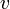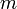# Cruise control

This page documents the cruise control system that is used as a running example throughout the text. A detailed description of the dynamics of this system are presented in Chapter 3 - Examples. This page contains a description of the system, including the models and commands used to generate some of the plots in the text.

## Introduction

Cruise control is the term used to describe a control system that regulates the speed of an automobile. Cruise control was commercially introduced in 1958 as an option on the Chrysler Imperial. The basic operation of a cruise controller is to sense the speed of the vehicle, compare this speed to a desired reference, and then accelerate or decelerate the car as required. The figure to the right shows a block diagram of this feedback system.

A simple control algorithm for controlling the speed is to use a "proportional plus integral" feedback. In this algorithm, we choose the amount of gas flowing to the engine based on both the error between the current and desired speed, and the integral of that error. The plot on the right shows the results of this feedback for a step change in the desired speed and a variety of different masses for the car (which might result from having a different number of passengers or towing a trailer). Notice that independent of the mass (which varies by a factor of 3), the steady state speed of the vehicle always approaches the desired speed and achieves that speed within approximately 5 seconds. Thus the performance of the system is robust with respect to this uncertainty.

## System model

The file {{{2}}} contains a simple model of a cruise controller for a car, depicted below.The system consists of a simple model of a vehicle (vehicle dynamics), with a proportional plus integral (PI) control law to track a step change in the car velocity. The commanded and actual variables are stored to the MATLAB workspace, along with the time vector. The vehicle model is defined in the file {{{2}}} and corresponds to the model described in Chapter 3 - Examples. Its dynamics are given bywhereis the vehicle velocity,is the mass of the vehicle,is the force applied by the engine andare the disturbance forces (aerodynamic drag, rolling friction and gravity). The engine force depends on the vehicle velocity and the throttle command,.

The model is initialized at 25 m/s (approximately 90 kph or 55 mph). The throttle command is saturated at the maximum torque of the engine. These dynamics are in the subsytem block labelled vehicle dynamics. The control law attempts to maintain the vehicle speed by applying a motor force that is the sum of two terms:

• a proportional gain that depends on the difference in speed between the commanded input and current vehicle speed
• an integral gain that depends on the integrated error of the commanded and current speed.

The commanded speed is set by a step input, which changes from 25 m/s to 30 m/s at t = 2 seconds. The integrator is initialized so that the initial input from the control law keeps the car at 25 m/s, the initial speed of the car. The gains for the control law are shown in the green (shaded) boxes. They can be set by double clicking on them and typing the new value in the dialog box. The default gains give a stable control law with some overshoot and a fairly long settling time. The simulation store three variables to the MATLAB workspace:

 ref the commanded (reference) velocity for the car vel the actual velocity of the car time the time vector for the simulaton

These variables are all stored as simple arrays, allowing them to be plotted using the plot command.